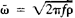# Figure of Equilibrium

The following article is from The Great Soviet Encyclopedia (1979). It might be outdated or ideologically biased.

## Figure of Equilibrium

a geometric configuration that can be assumed by a liquid mass in relative equilibrium. The term “relative equilibrium” is here taken to mean that the entire mass of the liquid is performing a steady motion such that the distances between the liquid’s particles remain constant. Two cases are considered: either the liquid moves in its own gravitational field alone or its motion is influenced by the attraction of external bodies in addition to the action of its own field. Two basic closely related problems are studied in the theory of figures of equilibrium: (1) the existence of particular figures of equilibrium of rotating liquids and (2) the stability of figures of equilibrium that are subjected to the influence of small disturbances.

Although the origins of the theory of figures of equilibrium date back to the 17th century, the theory remains far from completion. The most far-reaching results have been obtained by A. M. Liapunov and H. Poincaré. Liapunov was the first to construct a precise mathematical theory of the figures of equilibrium of both a rotating homogeneous liquid and a rotating heterogeneous liquid. In addition, he obtained various results in the theory of stability of the simple figures of equilibrium known as the Maclaurin spheroid and the Jacobi ellipsoid. Poincaré showed, in particular, that the relative equilibrium of a homogeneous liquid is possible only when the angular velocity of rotation ω is less than the quantity, where f is the gravitational constant, ρ is the density of the liquid, and ω̅ is what is called the Poincaré limit.

If no external forces are acting on a homogeneous, incompressible liquid mass that is at rest, the only figure of equilibrium of the mass is a sphere. The figures of equilibrium of a homogeneous liquid are in all cases symmetrical relative to the plane that passes through the liquid’s center of inertia and is perpendicular to the axis of rotation. Furthermore, any straight line parallel to the axis of rotation intersects the surface of the liquid at no more than two points.

The most thoroughly studied figures of equilibrium for a homogeneous incompressible rotating liquid are ellipsoidal: ellipsoids of rotation and triaxial ellipsoids. The families of surfaces formed by these figures of equilibrium constitute “linear” sequences that are continuously dependent on the magnitude of the angular velocity ω, which varies between zero and a value less than ω̅. Ellipsoidal figures of equilibrium are described mathematically by second-order algebraic surfaces. Poincaré provided an approximate solution to the problem of the existence of figures of equilibrium described by higher-order algebraic surfaces; a rigorous solution was given by Liapunov, who proved that there exist nearly ellipsoidal figures of equilibrium that are describable by algebraic surfaces of order greater than two. In this way, the problem of the stability of ellipsoidal figures for small deformations of the configuration was solved.

Of great practical importance is the theory of figures of equilibrium of a liquid that experiences the attraction of external forces. For the static model, where a liquid at rest is attracted by a sufficiently remote material point, it has been shown that there exist ellipsoidal figures of equilibrium that are prolate in the direction of the attracting point (tidal ellipsoids). An important role is played in astronomy by Roche’s problem and its generalizations. The problem establishes the existence of ellipsoidal or nearly ellipsoidal figures of equilibrium of a homogeneous rotating liquid mass that is attracted by a material point performing circular motion around the center of mass of the liquid at the same angular velocity. These mechanical models have provided a basis for the theory of tidal evolution, the theory of the shapes of stars in binary systems, and the theory of the figures of the planets. G. Darwin and J. Jeans developed an approximate theory of the stability of the figures of equilibrium of celestial bodies.

### REFERENCES

Subbotin, M. F. Kurs nebesnoi mekhaniki, vol. 3. Moscow-Leningrad, 1949.
Chandrasekhar, S. Ellipsoidal’nye figury ravnovesiia. Moscow, 1973. (Translated from English.)

E. A. GREBENIKOV